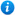* Watermarks do not appear on purchased resources

Preview resource: Click slides to enlarge

# Equivalent Fractions - Year 5

KS2 Maths Teaching Resources: Equivalent Fractions- Year 5

Year 5 maths programme of study - Number - fractions (including decimals and percentages):

• identify, name and write equivalent fractions of a given fraction, represented visually, including tenths and hundredths

In this teaching resource, pupils will learn how to identify, name and write equivalent fractions of a given fraction including tenths and hundredths as per the curriculum objective of the year 5 maths programme of study listed above.

This colourful and animated 62 slide PowerPoint presentation is suitable to use with all abilities and is also fully editable allowing teachers to adapt the resource to suit the individual teaching requirements of each class taught. Content includes:

• What are equivalent fractions?
• Identify equivalent fractions on a fraction wall activity and worksheet
• Identify equivalent fractions from diagrams activity and worksheet
• Identify and draw diagrams of equivalent fractions activity and worksheet
• How to calculate equivalent fractions explanation
• Calculating equivalent fractions activity and worksheets
• The 1/2, 1/4, 1/3, 1/5 and 1/10 family of equivalent fractions
• Match the pairs of equivalent fractions activity and worksheet
• Fraction slap game class activity
• Equivalent fractions activity and worksheet
• Equivalent fraction problems activity and worksheet
• Link to an equivalent fraction memory game

'Equivalent Fractions - Year 5' can be previewed in full by clicking on the PowerPoint images.

Our Price : £3.99 / 4 Credits## Get this resource as part of a bundle and save up to 72%## Year 5 Home Learning Pack - Maths and English

£19.99

By continuing to use the site, you agree to the use of cookies. You can change this and find out more by following this link